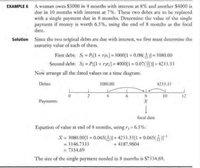# Interest (Payment)

#### girlie01

##### New member
Genie owes $3 000 in 4 months with simple interest at 8% and another$4 000 is due in 10 months with simple interest at 7%. These two debts are to be replaced with a single payment due in 8 months. Determine the value of the single payment if the money is worth 6.5% simple interest.

(I'm stuck on something)
I used the formula I=PRT for the two payments.
($3000)(0.08)(10) =$960
($4000)(0.07)(10) =$2800

#### girlie01

##### New member
Since the original debt are replaced by a new debt, the 7% and 8% are irrelevant.

A total of $7000, at 6.5%, is to be repaid in 8 months. What must the monthly payment be? using the formula, P= IRT, (7000)(0.065)(8), I got$3640. Just to clarify, this means that the 7% and 8% are distractions? Thank you

#### girlie01

##### New member
using the formula, P= IRT, (7000)(0.065)(8), I got $3640. Just to clarify, this means that the 7% and 8% are distractions? Thank you I meant, I= PRT, I = (7000)(0.065)(8/12) =$ 7 303.33, this is for the single payment...

#### jonah2.0

##### Full Member
Beer soaked recall and ramblings follow.
Genie owes $3 000 in 4 months with simple interest at 8% and another$4 000 is due in 10 months with simple interest at 7%. These two debts are to be replaced with a single payment due in 8 months. Determine the value of the single payment if the money is worth 6.5% simple interest.

(I'm stuck on something)
I used the formula I=PRT for the two payments.
($3000)(0.08)(10) =$960
($4000)(0.07)(10) =$2800
Then I add them together: \$3760
This is where I'm stuck. Do I use the same formula or find another one?
Your help is very much appreciated. Thanks
Equations of value involving simple interest.
Here's the very page from which that example problem was taken from.
Hopefully, you'll be able to do other similar exercises.•Subhotosh Khan and girlie01

#### girlie01

##### New member
Beer soaked recall and ramblings follow.

Equations of value involving simple interest.
Here's the very page from which that example problem was taken from.
Hopefully, you'll be able to do other similar exercises.
View attachment 26519
Yesss. I get it now. Thank you for this!